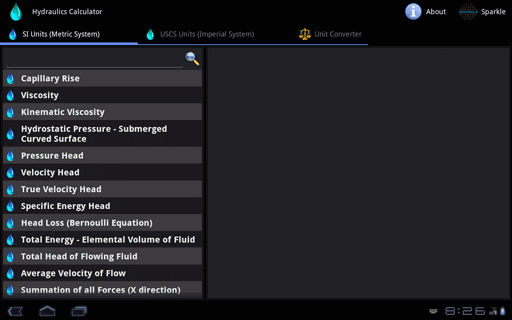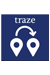### Hydraulics Calculator for Android Tablet##### Summary:
126 Calculators and Converters related to Hydraulics and WaterWorks. Available in both Metric and Imperial Units with Formulas. Automatic Calculation with Accurate Conversion.
1.0
Android
##### Category:
Education,Calculator
\$3.99
##### Requiements:
Works with all Android Tablets
##### Overview

Hydraulics Calculator contains 126 Calculators and Converters, that can quickly and easily calculate and convert different Hydraulics, WaterWorks and Civil Engineering parameters. Automatic & Accurate Calculations and Conversions with every Unit and Value Changes. Available in Imperial and Metric Units. Most Comprehensive Hydraulics & WaterWorks Calculator.Available in Metric and Imperial Units

Hydraulics Calculator contains following 94 Calculators:

• Capillary Rise
• Viscosity
• Kinematic Viscosity
• Hydrostatic Pressure - Submerged Curved Surface
• Total Energy - Elemental Volume of Fluid
• Total Head of Flowing Fluid
• Average Velocity of Flow
• Summation of all Forces (X direction)

• Froude Number
• Reynolds Number
• Weber Number
• Head Loss due to Friction
• Head Loss due to Friction (Darcy-Weisbach Formula)
• Chezy Velocity
• Chezy Coefficient

• Manning Flow Velocity
• Manning Flow Velocity using Pipe Diameter
• Flow Rate (Manning Formula)
• Head Loss due to Friction (Manning Formula)
• Pipe Diameter (Manning Formula)

• Mean Flow Velocity (Hazen-Williams Equation)
• Mean Flow Velocity - Pipes Flowing Full (Hazen-Williams Equation)
• Fluid Flow Rate (Hazen-Williams Equation)
• Head Loss due to Friction (Hazen-Williams Equation)
• Pipe Diameter (Hazen-Williams Equation)

• Head Loss across a sudden enlargement of Pipe Diameter (Bernoulli Equation)
• Head Loss across a sudden enlargement of Pipe Diameter (Archer Equation)
• Head Loss across a sudden contraction of Pipe
• Head Loss across a sudden contraction of Pipe (Brightmore Equation)
• Head Loss - Pipe Fittings

• Loss Coefficient
• Orifice Flow Rate
• Coefficient of Contraction
• Initial Velocity of Fluid Jets
• Gage Pressure at Tube Entrance (Conical Tubes)
• Velocity of Wave in a Pipe (Water Hammer)
• Internal Pressure in Pipe
• Stress on Pipe Material
• Section Factor
• Critical Depth of Flow - Open Channel Flow

• Force - Hydraulic Jump
• Rate of Change of Momentum - Hydraulic Jump
• Head Loss - Hydraulic Jump
• Stress on Pipe due to Temperature Change
• Movement in Length of Pipe due to Temperature Change
• Resultant Force on Bend in Pipe

• Elevation Difference between Headwater and Tailwater (Entrance and Exit Submerged)
• Velocity of Flow in Culvert (Entrance and Exit Submerged)
• Elevation Difference between Headwater and Tailwater (Entrance Submerged/Unsubmerged & Free Exit)
• Velocity of Flow in Culvert (Entrance Submerged/Unsubmerged & Free Exit)

• Flow Rate - Open Channel Flow
• Average Velocity - Rectangular Channels
• Critical Depth - Rectangular Channels
• Flow Rate - Rectangular Channels
• Discharge of Fluid per Unit Length - Rectangular Channels
• Minimum Specific Energy - Rectangular Channels

• Average Velocity - Triangular Channels
• Critical Depth - Triangular Channels
• Flow Rate - Triangular Channels

• Average Velocity - Parabolic Channels
• Critical Depth - Parabolic Channels
• Flow Rate - Parabolic Channels

• Average Velocity - Trapezoidal Channels
• Critical Depth - Trapezoidal Channels
• Flow Rate - Trapezoidal Channels
• Minimum Specific Energy - Trapezoidal Channels

• Area of Cross Section - Circular Channels
• Critical Depth - Circular Channels
• Top Width - Circular Channels
• Flow Rate - Circular Channels

• Flow Rate - Rectangular Weir
• Flow Rate - Triangular Weir (Notch Angle of 90 degrees)
• Flow Rate - Triangular Weir (Notch Angle of 60 degrees)
• Flow Rate - Triangular Weir (Notch Angle of 30 degrees)
• Flow Rate - Trapezoidal Weir
• Flow Rate - Broad Crested Weir

• Quantity of Sediment transported as Bed Load (Schoklitsch Formula)
• Evaporation Rate (Meyer Equation)
• Wind Factor
• Peak Discharge (Rational Formula)
• Rainfall Intensity (Steel Formula)

• Flow Rate - Groundwater (Darcy's Law)
• Fire Demand Rate
• Flow Rate - Gravity Well (Dupuit Formula)
• Flow Rate - Artesian Well
• Economic Pipe Diameter
• Flow Rate - Venturi Flow Meter
• Power obtained from Water Flow
Hydraulics Calculator contains following 32 Converters:

• Acceleration
• Angle
• Area
• Density
• Energy/Work
• Flow Rate (Mass)
• Flow Rate (Volume)
• Fluid
• Force
• Frequency
• Hardness
• Length
• Mass
• Metric Weight
• Metrology
• Moment of Force
• Moment of Inertia
• Prefixes
• Pressure
• Specific Heat Capacity
• Specific Volume
• Temperature
• Thermal Conductivity
• Thermal Expansion
• Time
• Torque
• Velocity
• Viscosity (Dynamic)
• Viscosity (Oil & Water)
• Viscosity (Kinematic)
• Volume
Key Features:
• Complete coverage of calculators and converters in Hydraulics, WaterWorks and Civil Engineering Parameters.
• Automatic Calculation & Conversion of the Output with respect to changes in the Input/Options/Units.
• Formulas are provided for each calculator.
• Values of Higher Order can also be calculated.
• Extremely Accurate Calculations and Conversions.
• Professionally and Newly designed user-interface that speeds up Data Entry, Easy Viewing and Calculation Speed.
Most Comprehensive Hydraulics & WaterWorks Calculator

•  Traze - reach ur friendsShare location with friends & view theirs. Navigate & make calls, all in one go. Traze enables two-way communication. Its never been easier to reach someone. More...Civil Engineering Pack for Android Tablet574 Calculators & Converters related to Civil, Beams, Columns, Piling, Concrete, Survey, Soil & Earthwork, Structural Engineering, Bridges, Highway & Road, Hydraulics and Timber. More...